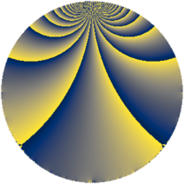# Properties

 Label 76.2.kLevel $76$ Weight $2$ Character orbit 76.k Rep. character $\chi_{76}(3,\cdot)$ Character field $\Q(\zeta_{18})$ Dimension $48$ Newform subspaces $1$ Sturm bound $20$ Trace bound $0$

# Related objects

## Defining parameters

 Level: $$N$$ $$=$$ $$76 = 2^{2} \cdot 19$$ Weight: $$k$$ $$=$$ $$2$$ Character orbit: $$[\chi]$$ $$=$$ 76.k (of order $$18$$ and degree $$6$$) Character conductor: $$\operatorname{cond}(\chi)$$ $$=$$ $$76$$ Character field: $$\Q(\zeta_{18})$$ Newform subspaces: $$1$$ Sturm bound: $$20$$ Trace bound: $$0$$

## Dimensions

The following table gives the dimensions of various subspaces of $$M_{2}(76, [\chi])$$.

Total New Old
Modular forms 72 72 0
Cusp forms 48 48 0
Eisenstein series 24 24 0

## Trace form

 $$48 q - 6 q^{2} - 12 q^{5} - 12 q^{6} - 9 q^{8} - 18 q^{9} + O(q^{10})$$ $$48 q - 6 q^{2} - 12 q^{5} - 12 q^{6} - 9 q^{8} - 18 q^{9} - 3 q^{10} - 9 q^{12} - 3 q^{14} - 12 q^{17} - 42 q^{20} - 18 q^{21} - 12 q^{22} + 24 q^{24} - 12 q^{25} + 21 q^{26} - 12 q^{29} + 42 q^{30} + 9 q^{32} - 36 q^{33} + 87 q^{36} + 60 q^{38} + 6 q^{40} + 30 q^{41} + 3 q^{42} + 45 q^{44} - 6 q^{45} + 36 q^{46} + 45 q^{48} - 18 q^{49} + 18 q^{50} - 15 q^{52} - 24 q^{53} - 75 q^{54} - 12 q^{57} + 60 q^{58} + 6 q^{60} - 66 q^{62} - 45 q^{64} + 18 q^{65} - 42 q^{66} - 42 q^{68} + 126 q^{69} - 63 q^{70} - 78 q^{72} - 12 q^{73} - 105 q^{74} - 126 q^{76} - 36 q^{77} + 3 q^{78} - 3 q^{80} + 72 q^{81} - 111 q^{82} - 117 q^{84} + 108 q^{85} - 24 q^{86} - 81 q^{88} - 18 q^{90} + 36 q^{92} + 30 q^{93} - 66 q^{96} - 6 q^{97} + 39 q^{98} + O(q^{100})$$

## Decomposition of $$S_{2}^{\mathrm{new}}(76, [\chi])$$ into newform subspaces

Label Dim $A$ Field CM Traces $q$-expansion
$a_{2}$ $a_{3}$ $a_{5}$ $a_{7}$
76.2.k.a $48$ $0.607$ None $$-6$$ $$0$$ $$-12$$ $$0$$